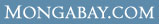# WHAT ARE THE AVERAGE Wages & salaries for Computer and mathematical occupations in Sumter, SC ?

 Overview Every year U.S. Department of Labor's Bureau of Labor Statistics releases employment and wage figures. This data was released in May 2006 and covers Wages & salaries for Computer and mathematical occupations in Sumter, SC Computer and mathematical occupations (Occupation [OCC] code: 15-0000) in Sumter, SC (BLS Area code: 44940 ) earned an average wage of \$23.48 per hour and an average annual salary of \$48,840 The BLS estimates that in Sumter, SC there were around 180 employed in the field of Computer and mathematical occupations.

Industry Comparison
Industry comparison
Computer and mathematical occupations in Sumter, SC earned 29% less than average for Computer and mathematical occupations in the United States.

Region/area/city comparison
Computer and mathematical occupations in Sumter, SC earned 63% more than the average worker in Sumter, SC.

State salary comparison
Computer and mathematical occupations in Sumter, SC earned 27% more than the average worker in South Carolina.

National salary comparison
Computer and mathematical occupations in Sumter, SC earned 25% more than the average worker in the United States.

Wage and salary details
More detailed employment information for Computer and mathematical occupations in Sumter, SC

Hourly wage data for Computer and mathematical occupations in Sumter, SC

 Mean hourly wage: \$23.48 Tenth (10th) percentile hourly wage: \$12.31 Twenty-fifth (25th) percentile hourly wage: \$18.18 Fiftieth (50th) percentile wage [median] hourly wage: \$24.23 Seventy-fifth (75th) percentile hourly wage: \$28.58 Ninetieth (90th) percentile hourly wage: \$34.11

Salary figures for Computer and mathematical occupations in Sumter, SC

 Mean annual salary: \$48,840 Tenth (10th) percentile annual salary: \$25,600 Twenty-fifth (25th) percentile annual salary: \$37,810 Fiftieth (50th) percentile wage [median] annual salary: \$50,390 Seventy-fifth (75th) percentile annual salary: \$59,450 Ninetieth (90th) percentile annual salary: \$70,960

Indexwhat's new | rainforests home | help support the site | madagascar | search | about | contact